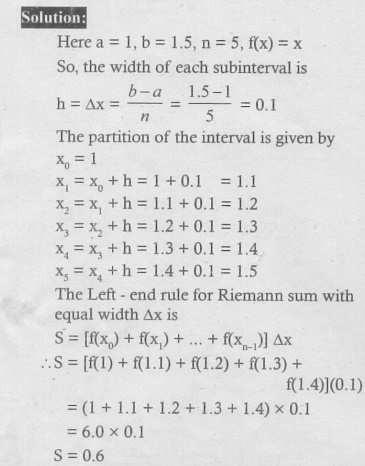Home | | Maths 12th Std | Exercise 9.1: Definite Integral as the Limit of a Sum

# Exercise 9.1: Definite Integral as the Limit of a Sum

Maths Book back answers and solution for Exercise questions - Mathematics : Applications of Integration: Definite Integral as the Limit of a Sum - Exercise Problem Questions with Answer, Solution

EXERCISE 9.1

1. Find an approximate value of 1.5Ōł½1 xdx by applying the left-end rule with the partition {1.1, 1.2, 1.3, 1.4, 1.5}.2. Find an approximate value of 1.5Ōł½1 x2 dx by applying the right-end rule with the partition {1.1, 1.2, 1.3, 1.4, 1.5}.3. Find an approximate value of  1.5Ōł½( 2 ŌłÆ x dx by applying the mid-point rule with the partition {1.1, 1.2, 1.3, 1.4, 1.5}.